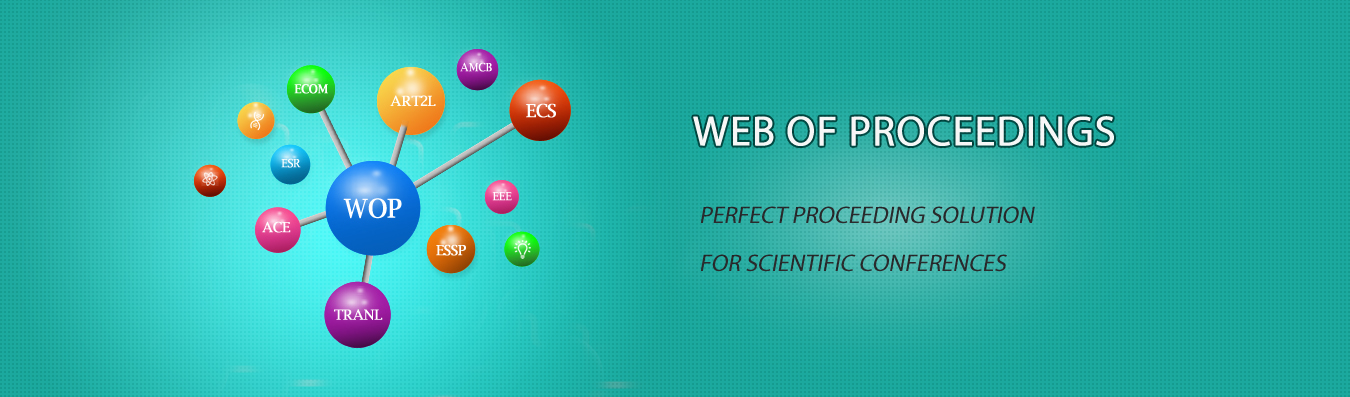The best way to conference proceedings by Francis Academic Press### Error Bounds for Linear Complementarity Problems of S-Nekrasov Matrices and B-S-Nekrasov Matrices

DOI: 10.25236/icssae.2019.071

Yanyan Li

Yanyan Li

#### Abstract

First, the estimation of the error bounds of the S-Nekrasov matrix is studied. Using the properties of S-Nekrasov matrices and , The properties of inequalities, And the estimators of the upper bound of infinite norm for the inverse matrix of matrix, The estimation of the error bounds of the matrix is obtained, Further on this basis the estimation formula for the error bounds of the B-S-Nekrasov matrix is also obtained.

#### Keywords

Linear complementarity problems; Error bounds; S-Nekrasov matrices; B-S-Nekrasov matrices